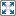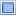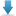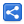# TypeScript - Applying Mixins

[Last Updated: Aug 11, 2020]

Mixin is the process of combining multiple classes to a single target class. It is intended to overcome the limitations of single inheritance model of JavaScript.

In TypeScript, the target class combines the multiple classes by using implements keyword. It is needed to maintain the TypeScript strong typing for the target mixin class.

We also need a generic helper function that applies the actual mixing for us. This function runs through the properties of each of the mixins and copy them over to the target of the mixins.

# Example

```class Shape {
draw(): void {
console.log("shape is drawing");
}
}

class Component {
display(): void {
console.log("component is displaying");
}
}

class Rectangle implements Shape, Component {
w: number;
h: number;

constructor(w, h) {
this.w = w;
this.h = h;
}

area(): number {
return this.w * this.h;
}

//just provide the empty implementation which will be replaced by the mixins helper function
//same as display: () => void
display(): void {
}

//same as draw: () => void
draw(): void {
}
}

//applying mixing which iterates through properties of baseCtors classes  and copy them to the target class (derivedCtor)
function applyMixins(derivedCtor: any, baseCtors: any[]) {
baseCtors.forEach(baseCtor => {
Object.getOwnPropertyNames(baseCtor.prototype).forEach(name => {
Object.defineProperty(derivedCtor.prototype, name,
Object.getOwnPropertyDescriptor(baseCtor.prototype, name));
});
});
}

applyMixins(Rectangle, [Shape, Component]);

let rectangle: Rectangle = new Rectangle(4, 3);
rectangle.draw();
rectangle.display();
let area = rectangle.area();
console.log(area);```

#### Output

`shape is drawingcomponent is displaying12`

### Compiled JavaScript

```class Shape {
draw() {
console.log("shape is drawing");
}
}
class Component {
display() {
console.log("component is displaying");
}
}
class Rectangle {
constructor(w, h) {
this.w = w;
this.h = h;
}
area() {
return this.w * this.h;
}
//just provide the empty implementation which will be replaced by the mixins helper function
//same as display: () => void
display() {
}
//same as draw: () => void
draw() {
}
}
//applying mixing which iterates through properties of baseCtors classes  and copy them to the target class (derivedCtor)
function applyMixins(derivedCtor, baseCtors) {
baseCtors.forEach(baseCtor => {
Object.getOwnPropertyNames(baseCtor.prototype).forEach(name => {
Object.defineProperty(derivedCtor.prototype, name, Object.getOwnPropertyDescriptor(baseCtor.prototype, name));
});
});
}
applyMixins(Rectangle, [Shape, Component]);
let rectangle = new Rectangle(4, 3);
rectangle.draw();
rectangle.display();
let area = rectangle.area();
console.log(area);
```

## Example Project

Dependencies and Technologies Used:

• TypeScript 3.4.5
 TypeScript - Applying MixinsSelect AllDownload• typescript-mixins-examples
• MixinExample.ts

 Share